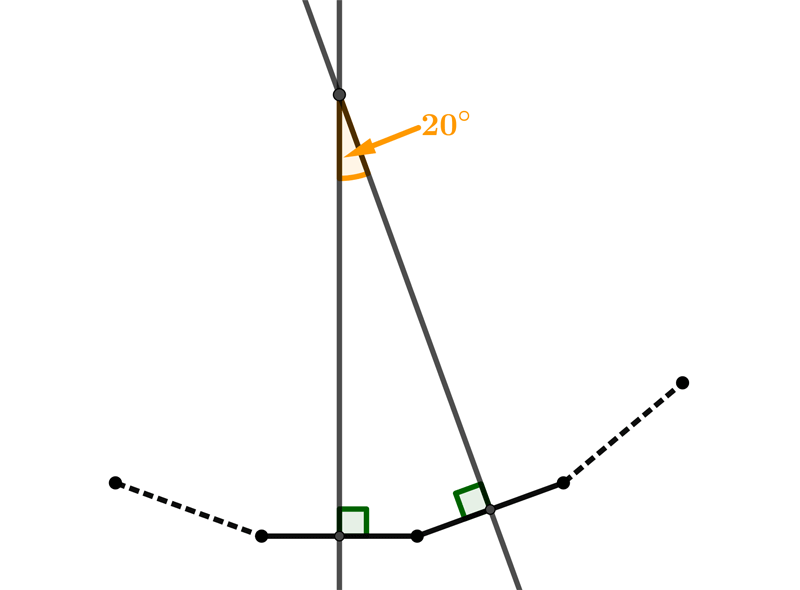Math Calculators, Lessons and Formulas

It is time to solve your math problem

mathportal.org
• Geometry
• Polygons
• Angles of regular polygons

# Angles of regular polygons

ans:
syntax error
C
DEL
ANS
±
(
)
÷
×
7
8
9
4
5
6
+
1
2
3
=
0
.
auto next question
calculator
•  Question 1: 1 pts The size of the exterior angles of the regular polygon shown on the picture is $220^{\circ}.$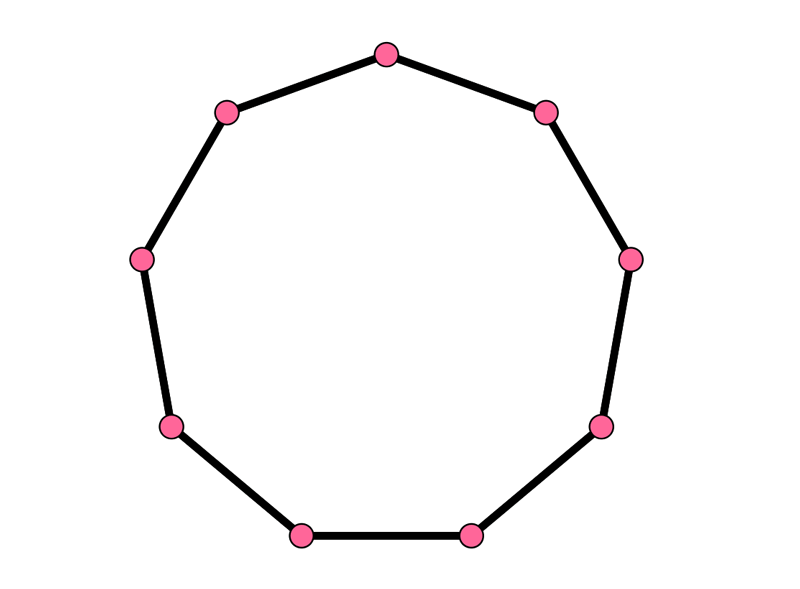•  Question 2: 1 pts The size of the interior angles of the regular polygon shown on the picture is $160^{\circ}.$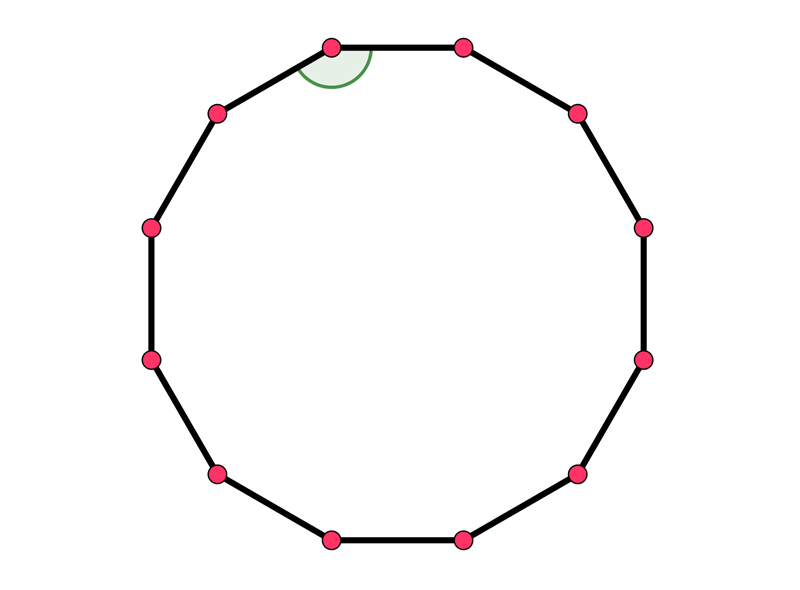•  Question 3: 1 pts Find the size of an interior angle of a regular polygon with $20$ sides.
•  Question 4: 1 pts Which regular polygons have the exterior angles of $9$ degrees?
 $36-$ sided polygon $38-$ sided polygon $40-$ sided polygon $42-$ sided polygon
•  Question 5: 2 pts Here is the vertex of a regular polygon. How many sides does it have?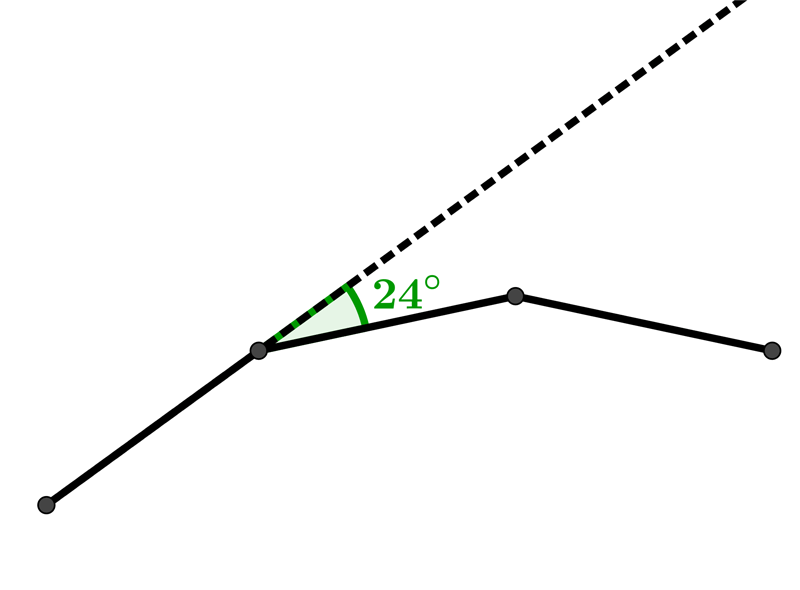$9$ $11$ $13$ $15$
•  Question 6: 2 pts The diagrams shows a regular decagon. Find the measure of angle marked $x.$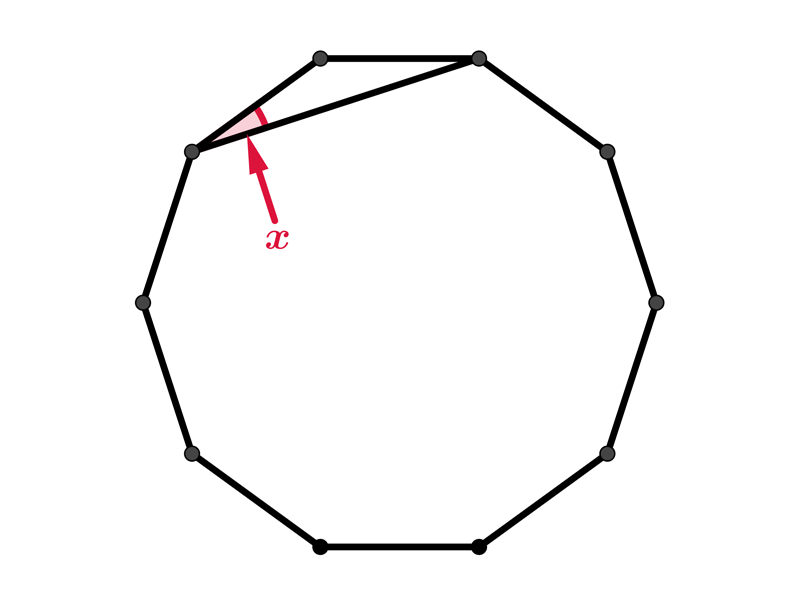$x=18^{\circ}$ $x=20^{\circ}$ $x=26^{\circ}$ $x=56^{\circ}$
•  Question 7: 2 pts The diagram shows a regular hexagon joined to a regular pentagon. The angles labelled $a, b$ and $c$ are equal $$a=108^{\circ}, b=120^{\circ} \mbox{ and } c=132^{\circ}.$$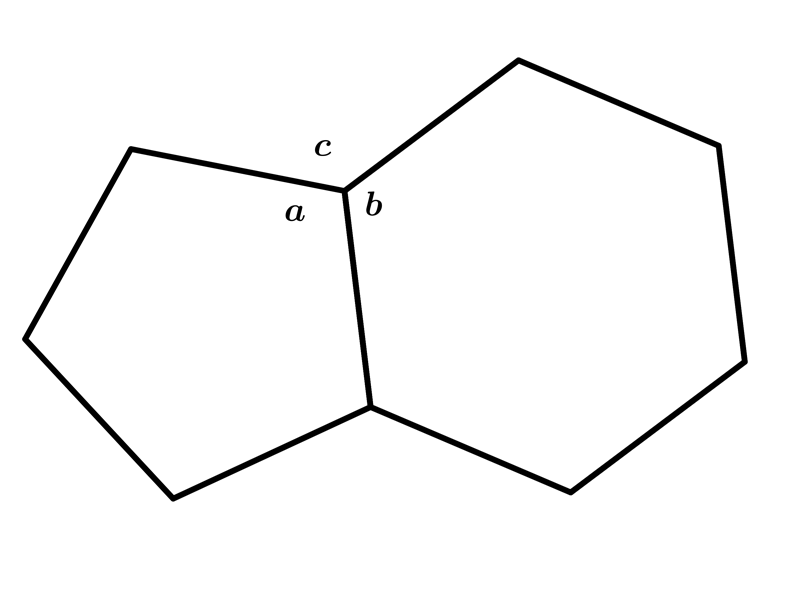•  Question 8: 2 pts One exterior angle of the regular polygon is $20$ degrees. Find the sum of its interior angles.
 $1620^{\circ}$ $2160^{\circ}$ $2700^{\circ}$ $2880^{\circ}$
•  Question 9: 3 pts Rectangular flowerbeds are built on each side of a fishpond in the shape of a regular octagon. What is the measure of the angle $x$, between two consecutive flowerbeds?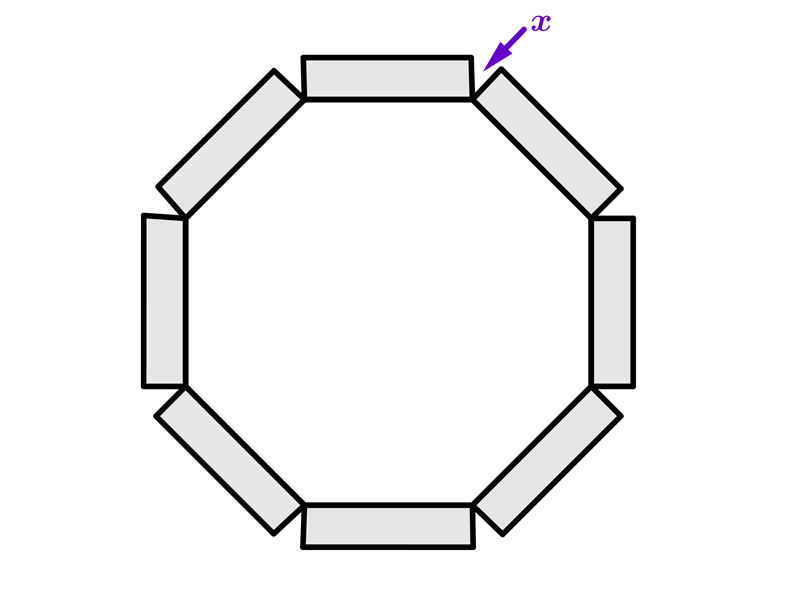$20^{\circ}$ $30^{\circ}$ $40^{\circ}$ $45^{\circ}$
•  Question 10: 3 pts The regular polygon shown on the picture have $18$ sides.